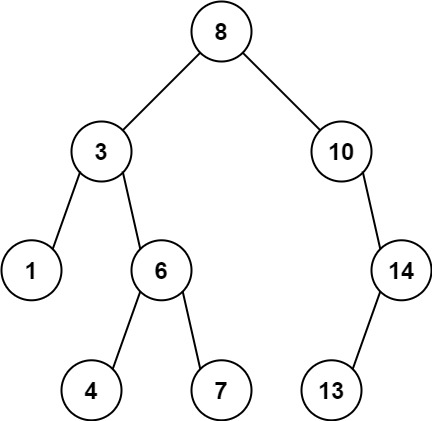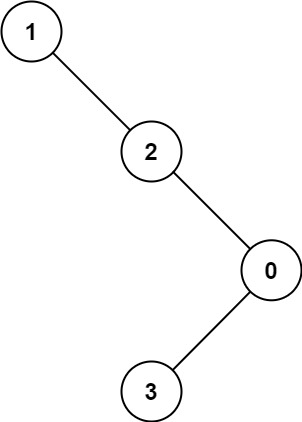# GeetCode Hub

Given the `root` of a binary tree, find the maximum value `V` for which there exist different nodes `A` and `B` where `V = |A.val - B.val|` and `A` is an ancestor of `B`.

A node `A` is an ancestor of `B` if either: any child of `A` is equal to `B`, or any child of `A` is an ancestor of `B`.

Example 1:```Input: root = [8,3,10,1,6,null,14,null,null,4,7,13]
Output: 7
Explanation: We have various ancestor-node differences, some of which are given below :
|8 - 3| = 5
|3 - 7| = 4
|8 - 1| = 7
|10 - 13| = 3
Among all possible differences, the maximum value of 7 is obtained by |8 - 1| = 7.```

Example 2:```Input: root = [1,null,2,null,0,3]
Output: 3
```

Constraints:

• The number of nodes in the tree is in the range `[2, 5000]`.
• `0 <= Node.val <= 105`

/** * Definition for a binary tree node. * public class TreeNode { * int val; * TreeNode left; * TreeNode right; * TreeNode() {} * TreeNode(int val) { this.val = val; } * TreeNode(int val, TreeNode left, TreeNode right) { * this.val = val; * this.left = left; * this.right = right; * } * } */ class Solution { public int maxAncestorDiff(TreeNode root) { } }